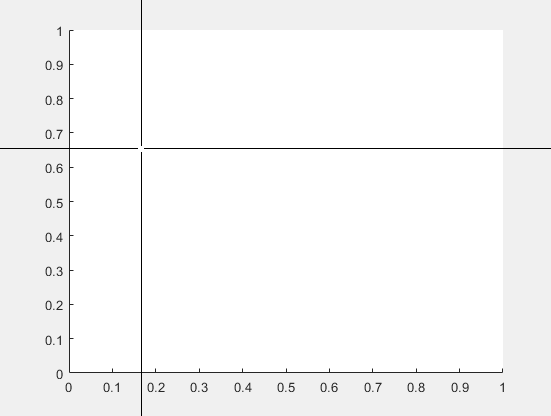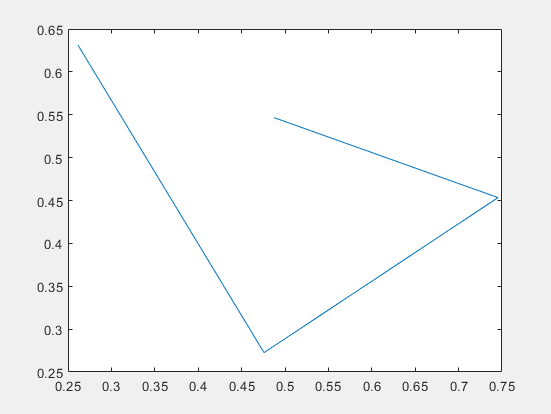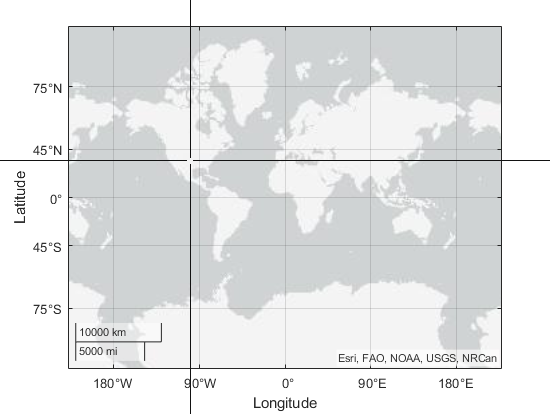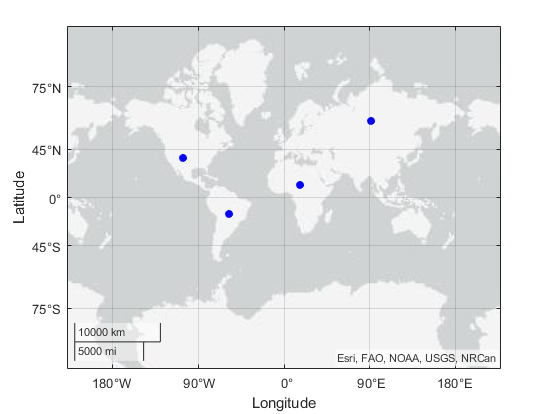# ginput

## 语法

``[x,y] = ginput(n)``
``[x,y] = ginput``
``[x,y,button] = ginput(___)``

## 说明

``[x,y] = ginput(n)` 可用于标识笛卡尔坐标区、极坐标区或地理坐标区内 `n` 个点的坐标。要选择一个点，请将光标移至所需位置，然后按下鼠标按键或键盘上的键。在选中全部 `n` 个点之前，按 Return 键可停止选择。MATLAB® 返回所选点的坐标。如果没有坐标区，则调用 `ginput` 会创建一个笛卡尔坐标区对象。`

``[x,y] = ginput` 可用于选择无限多个点，直到您按 Return 键为止。`

``[x,y,button] = ginput(___)` 还返回用于选择每个点的鼠标按键或键盘上的键。`

## 示例

`[x,y] = ginput(4)````x = 0.3699 0.4425 0.5450 0.6130 y = 0.6690 0.5605 0.4719 0.6025```

`plot(x,y);````[x,y,button] = ginput(5); button ```
```button = 3 104 32 51 82```

```geoaxes [lat,lon] = ginput(4);``````hold on geolimits('manual') geoscatter(lat,lon,'filled','b')```## 输出参数

• 对于笛卡尔坐标区，值为 `x` 坐标。

• 对于极坐标区，值为 theta 角度。theta 角度在范围 `[tmin, tmin+2*pi]` 内，其中 `tmin` 是极坐标区的 `ThetaLim` 向量的第一个值。角度是在 `ThetaDir` 属性指定的方向上从零角度位置开始测量的。零角度的位置由 `ThetaZeroLocation` 属性指定。

• 对于地理坐标区，值是纬度。正值对应于北纬，负值对应于南纬。

• 对于笛卡尔坐标区，值为 `y` 坐标。

• 对于极坐标区，值是半径。每个半径值都大于或等于 `rmin`，其中 `rmin` 是极坐标区的 `RLim` 向量中的第一个值。

• 对于地理坐标区，值是经度。正值对应于原点以东的位置，负值对应于原点以西的位置。

`[~,~,button] = ginput`
```button = 2 3 1```